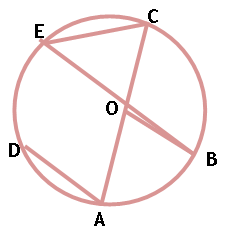Maths / Circles / Equal Chords of a Circle Subtend Equal Angle at the Centre

QUESTION

 If   then which of the following relations is true ?​​​OPTIONS A. B. C. D.
Right Option : A

EXPLANATION
Explain TypeExplanation Content
Text
 As   thenAB = BC then as equal chords subtend equal angle at the center so equal angle at circumference.​​​TestimonialsStudent Feedback on Methodology - Aman Kumar Shrivastava C/o ABHYAS Academy
10th
One of the best institutes to develope a child interest in studies.Provides SST and English knowledge also unlike other institutes. Teachers are co operative and friendly online tests andPPT develope practical knowledge also.